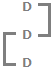Verbal Reasoning - Arithmetic Reasoning - Discussion

Discussion :: Arithmetic Reasoning - Section 1 (Q.No.35)

35.

What is the smallest number of ducks that could swim in this formation - two ducks in front of a duck, two ducks behind a duck and a duck between two ducks ?

 [A]. 3 [B]. 5 [C]. 7 [D]. 9

Explanation:

Clearly, the smallest such number is 3.Three ducks can be arranged as shown above to satisfy all the three given conditions.